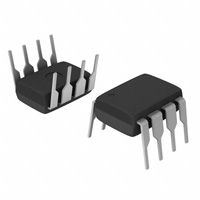< id="6e2kg">< id="6e2kg">< id="6e2kg">< id="6e2kg">< id="6e2kg">< id="6e2kg">< id="6e2kg">< id="6e2kg">< id="6e2kg">< id="6e2kg">< id="6e2kg">< id="6e2kg">< id="6e2kg">< id="6e2kg">< id="6e2kg">< id="6e2kg">< id="6e2kg">< id="6e2kg">< id="6e2kg">< id="6e2kg">< id="6e2kg">< id="6e2kg">< id="6e2kg">< id="6e2kg">< id="6e2kg">< id="6e2kg">< id="6e2kg">< id="6e2kg">< id="6e2kg">< id="6e2kg">< id="6e2kg">< id="6e2kg">< id="6e2kg">< id="6e2kg">< id="6e2kg">< id="6e2kg">< id="6e2kg">< id="6e2kg">< id="6e2kg">< id="6e2kg">< id="6e2kg">< id="6e2kg">< id="6e2kg">< id="6e2kg">< id="6e2kg">< id="6e2kg">< id="6e2kg">< id="6e2kg">< id="6e2kg">< id="6e2kg"> < id="6e2kg">< id="6e2kg">< id="6e2kg">< id="6e2kg">< id="6e2kg">< id="6e2kg">< id="6e2kg">< id="6e2kg">< id="6e2kg">< id="6e2kg">< id="6e2kg">< id="6e2kg">< id="6e2kg">< id="6e2kg">< id="6e2kg">< id="6e2kg">< id="6e2kg">< id="6e2kg">< id="6e2kg">< id="6e2kg">< id="6e2kg">< id="6e2kg">< id="6e2kg">< id="6e2kg">< id="6e2kg">< id="6e2kg">< id="6e2kg">< id="6e2kg">< id="6e2kg">< id="6e2kg">< id="6e2kg">< id="6e2kg">< id="6e2kg">< id="6e2kg">< id="6e2kg">< id="6e2kg">< id="6e2kg">< id="6e2kg">< id="6e2kg">< id="6e2kg">< id="6e2kg">< id="6e2kg">< id="6e2kg">< id="6e2kg">< id="6e2kg">< id="6e2kg">< id="6e2kg">< id="6e2kg">< id="6e2kg">< id="6e2kg"> < id="6e2kg">< id="6e2kg">< id="6e2kg">< id="6e2kg">< id="6e2kg">< id="6e2kg">< id="6e2kg">< id="6e2kg">< id="6e2kg">< id="6e2kg">< id="6e2kg">< id="6e2kg">< id="6e2kg">< id="6e2kg">< id="6e2kg">< id="6e2kg">< id="6e2kg">< id="6e2kg">< id="6e2kg">< id="6e2kg">< id="6e2kg">< id="6e2kg">< id="6e2kg">< id="6e2kg">< id="6e2kg">< id="6e2kg">< id="6e2kg">< id="6e2kg">< id="6e2kg">< id="6e2kg">< id="6e2kg">< id="6e2kg">< id="6e2kg">< id="6e2kg">< id="6e2kg">< id="6e2kg">< id="6e2kg">< id="6e2kg">< id="6e2kg">< id="6e2kg">< id="6e2kg">< id="6e2kg">< id="6e2kg">< id="6e2kg">< id="6e2kg">< id="6e2kg">< id="6e2kg">< id="6e2kg">< id="6e2kg">< id="6e2kg">型号： IR2101 厂商：International Rectifier 分类： 集成电路 ， PMIC - MOSFET，电桥驱动器 - 外部开关 描述： IC driver high/low side 8-dip PDF： 预览 报错 收藏 赞

Datasheet下载地址

 本地下载 >> 厂商下载 >>

IR2101的详细信息

Datasheets： 50 Integrated Circuits (ICs) PMIC - MOSFET, Bridge Drivers - External Switch - Tube High and Low Side, Independent Non-Inverting 160ns 210mA 1 2 600V 10 V ~ 20 V -40°C ~ 125°C Through Hole 8-DIP (0.300", 7.62mm) 8-DIP *IR2101
Data Sheet No. PD60043 Rev.O
( )
( )
&(PbF)
S
S
IR2101 /IR2102
HIGH AND LOW SIDE DRIVER
Features
Product Summary
? Floating channel designed for bootstrap operation
V
600V max.
130 mA / 270 mA
10 - 20V
OFFSET
Fully operational to +600V
Tolerant to negative transient voltage
dV/dt immune
? Gate drive supply range from 10 to 20V
? Undervoltage lockout
I +/-
O
V
OUT
? 3.3V, 5V, and 15V logic input compatible
? Matched propagation delay for both channels
t
(typ.)
160 & 150 ns
50 ns
on/off
? Outputs in phase with inputs (IR2101) or out of
Delay Matching
phase with inputs (IR2102)
Packages
Description
The IR2101(S)/IR2102(S) are high voltage, high speed
power MOSFET and IGBT drivers with independent
high and low side referenced output channels. Pro-
prietary HVIC and latch immune CMOS technologies
enable ruggedized monolithic construction. The logic
input is compatible with standard CMOS or LSTTL
IR2101S/IR2102S
IR2101/IR2102
output, down to 3.3V logic. The output drivers feature a high pulse current buffer stage designed for minimum
driver cross-conduction. The floating channel can be used to drive an N-channel power MOSFET or IGBT in
the high side configuration which operates up to 600 volts.
Typical Connection
up to 600V
VCC
VCC
VB
HO
VS
HIN
LIN
HIN
LIN
TO
COM
LO
IR2101
up to 600V
VCC
VCC
VB
HO
VS
HIN
LIN
HIN
LIN
TO
(Refer to Lead Assignments for correct pin
configuration). This/These diagram(s) show
electrical connections only. Please refer to
our Application Notes and DesignTips for
proper circuit board layout.
COM
LO
IR2102
www.irf.com
1